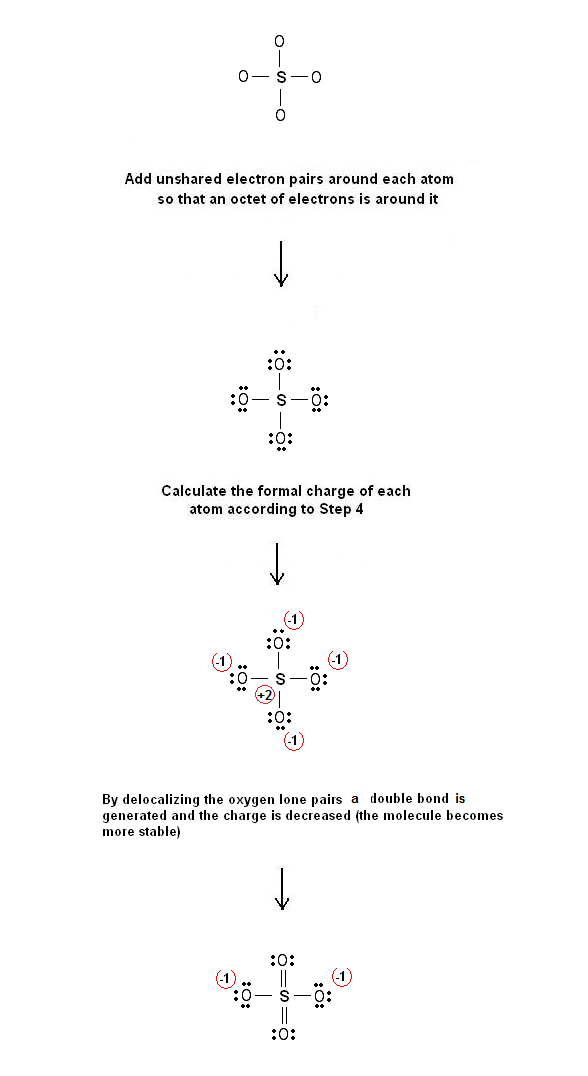Electron dot structure of the sulfate ion SO4-2 - #52 | Chemistry Net

# Electron dot structure of the sulfate ion SO4-2 - #52

A simple procedure for writing electron-dot Lewis Structures was given in a previous article entitled “Lewis Structures and the Octet Rule”.
Several worked examples relevant to this procedure were given in previous posts. Please see the Sitemap - Table of Contents (Lewis Electron Dot Structures)

Let us consider the case of SO4-2
The first description of the SO4-2 bonding in modern times proposed by G.N. Lewis in his paper "The Atom and the Molecule" published in 1911. He described the bonding in terms of  electron octets around each atom, that is no double bonds and a charge of +2 on the sulfur atom. Later Linus Pauling used valence bond theory to propose in his paper "The Modern Theory of Valency" that the most significant resonance structures had two π bonds involving d orbitals.

Step 1: Connect the atoms with single bonds:Fig. 1:  The SO4-2  atoms connected with single bonds

Step 2:  Calculate the # of electrons in π bonds (multiple bonds) using  formula (1):

Where n in this case is 5. Where V = (6 + 6 + 6 + 6 + 6) – (-2) = 32 , V is the number of valence electrons of the molecule.
Therefore, P = 6n + 2 – V = 6 * 5 + 2 – 32  = 0     So, there is no multiple bond in the molecule.

Step 3 & 4: The Lewis dot resonance structures of   SO4-2 are as follows:Fig. 2: Lewis dot structure of sulfate ion SO4-2

#### 1 comment:

1.Why you add those other bonds around S and how is that possible??